# Selina Solutions Concise Maths Class 7 Chapter 15: Triangles Exercise 15A

Selina Solutions Concise Maths Class 7 Chapter 15 Triangles Exercise 15A consists of problems on determining the angle of the given set of triangles. A triangle is a closed figure which has three sides and vertices. The main aim of creating the exercise wise solutions is to provide a strong foundation of fundamental concepts covered in this exercise. These concepts are important as it will be continued in further classes also. For a clear conceptual knowledge, students can access Selina Solutions Concise Maths Class 7 Chapter 15 Triangles Exercise 15A PDF, from the links which are available here.

## Selina Solutions Concise Maths Class 7 Chapter 15: Triangles Exercise 15A Download PDF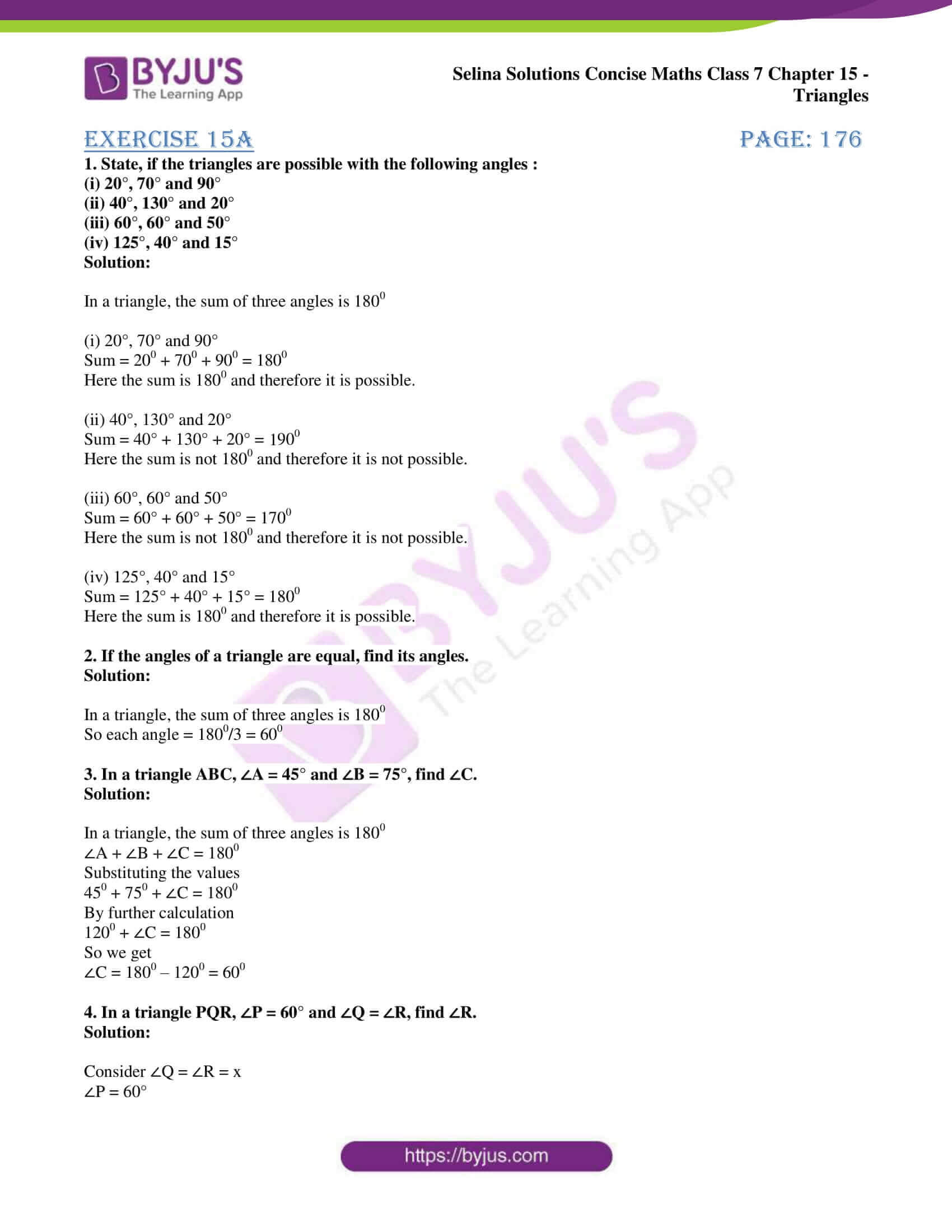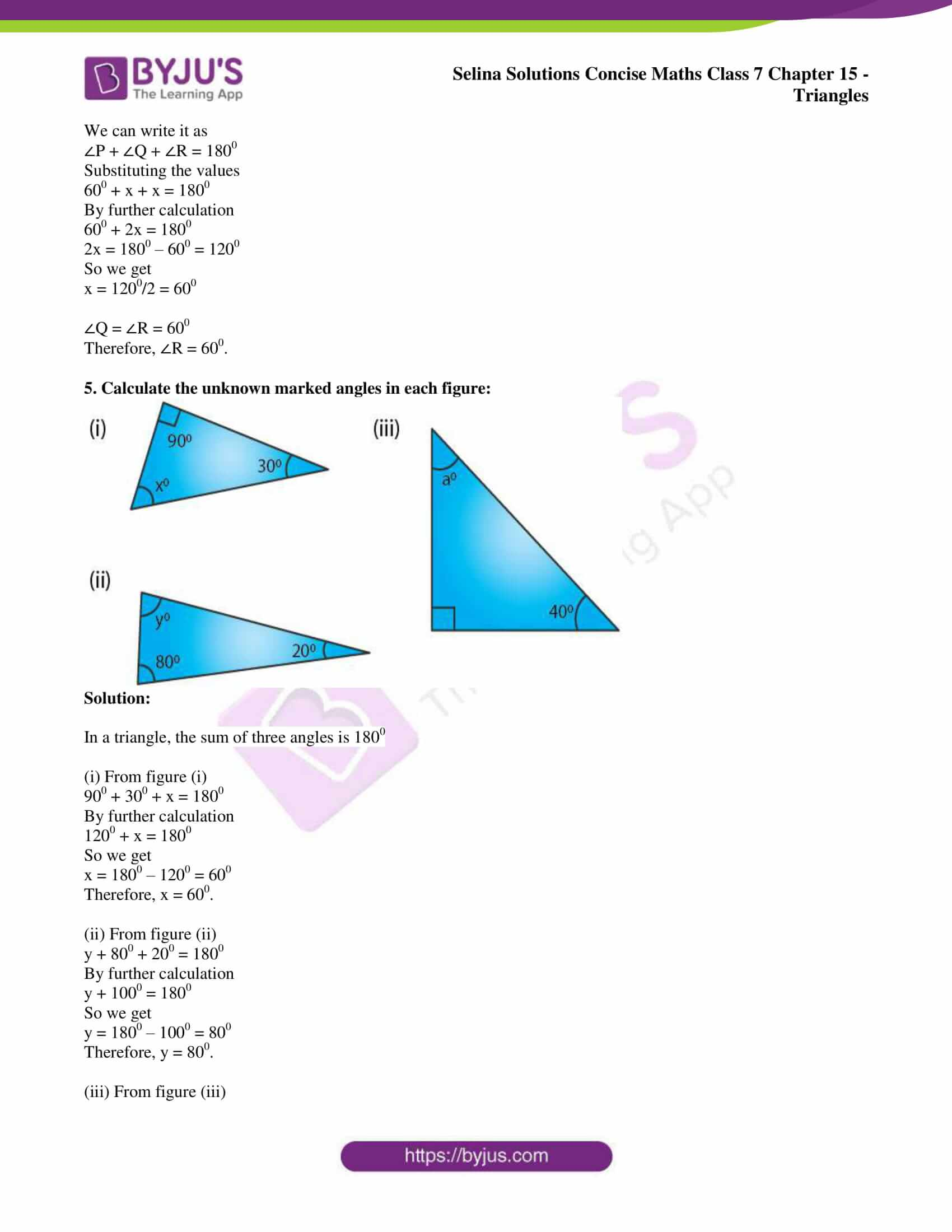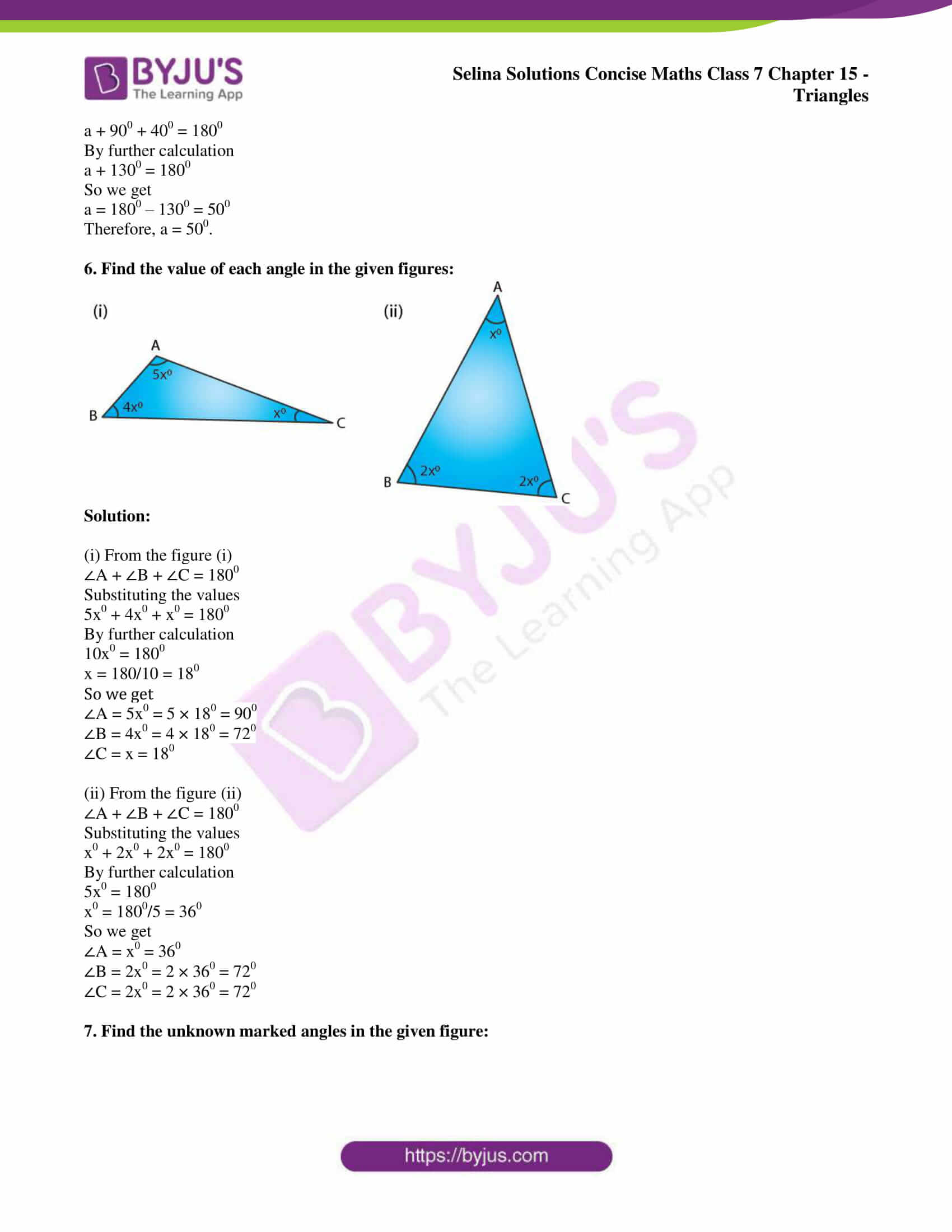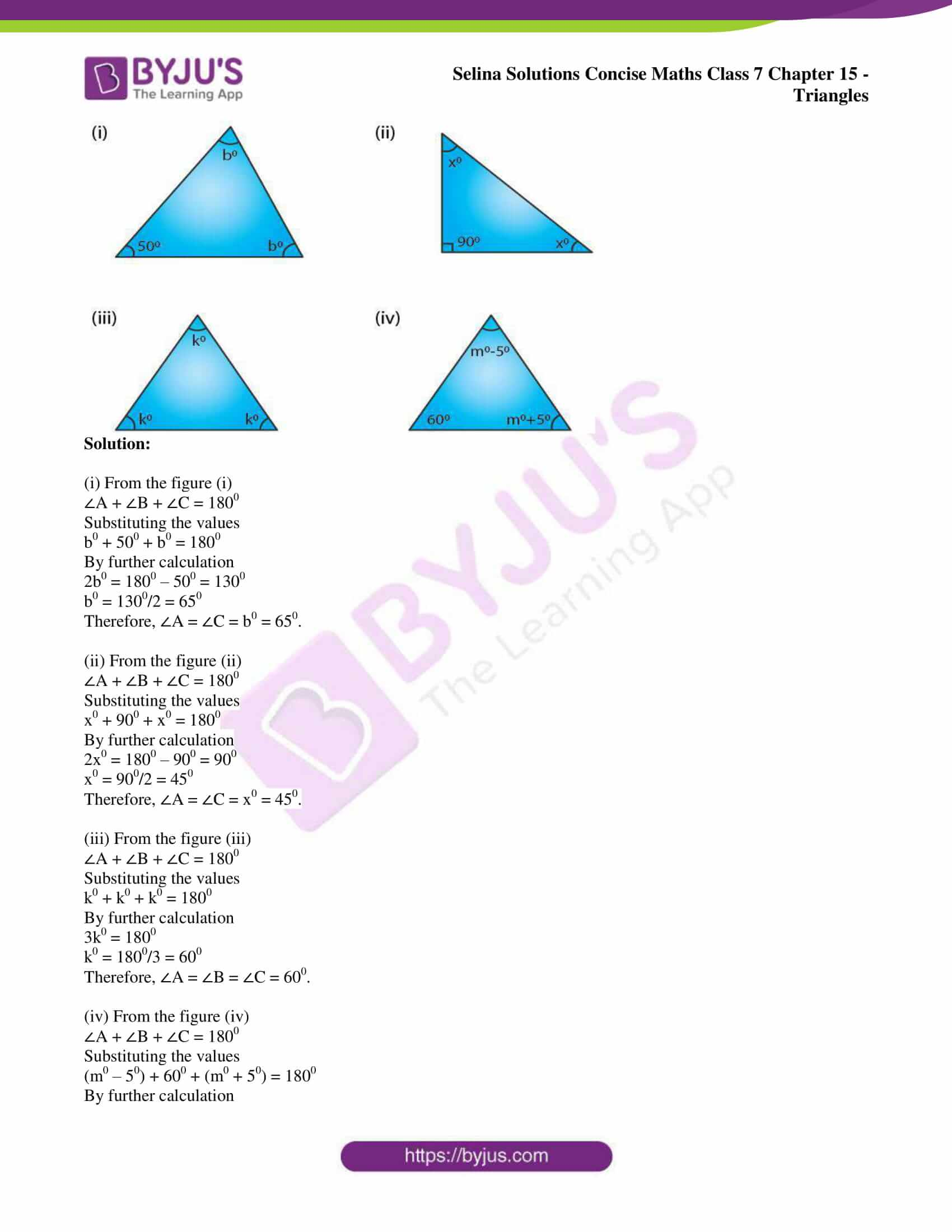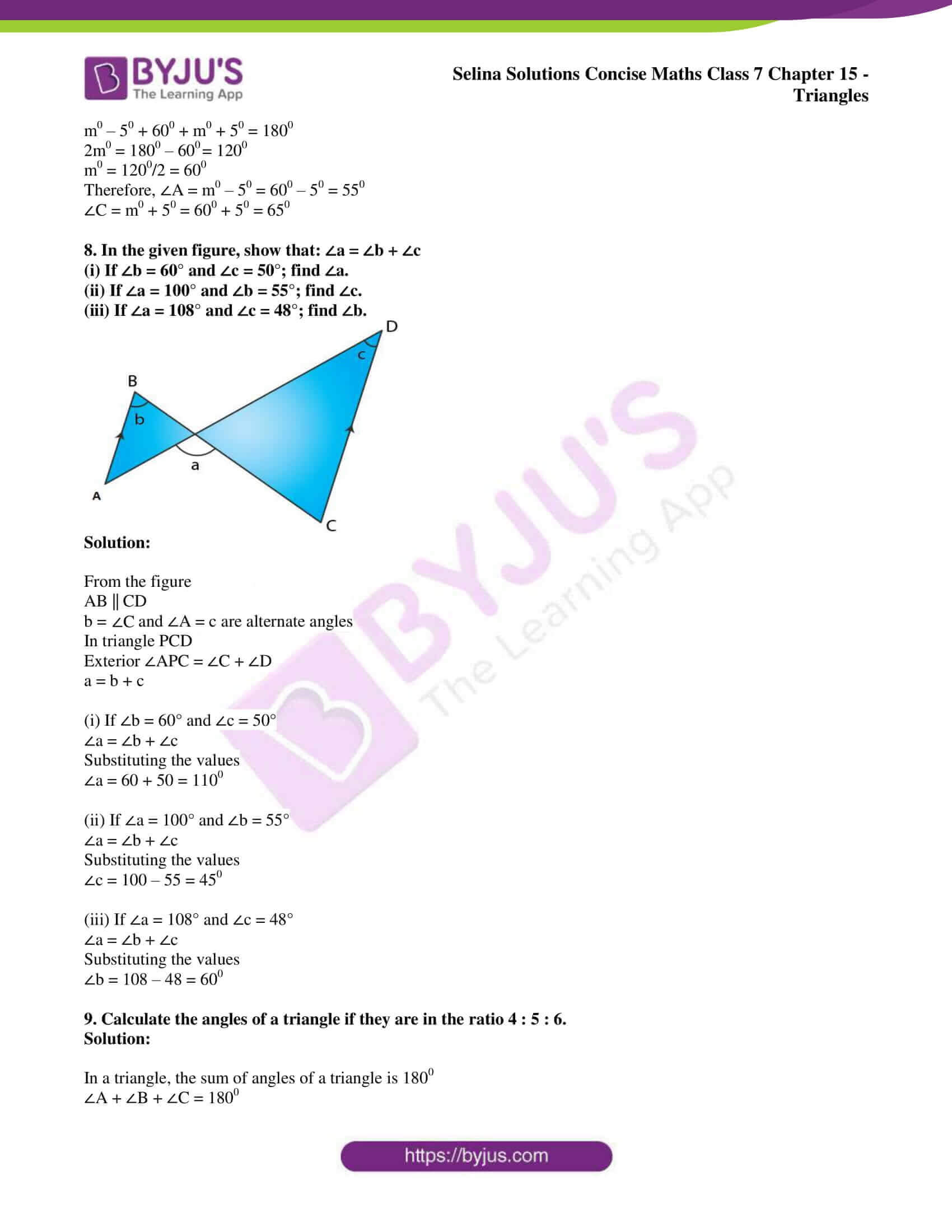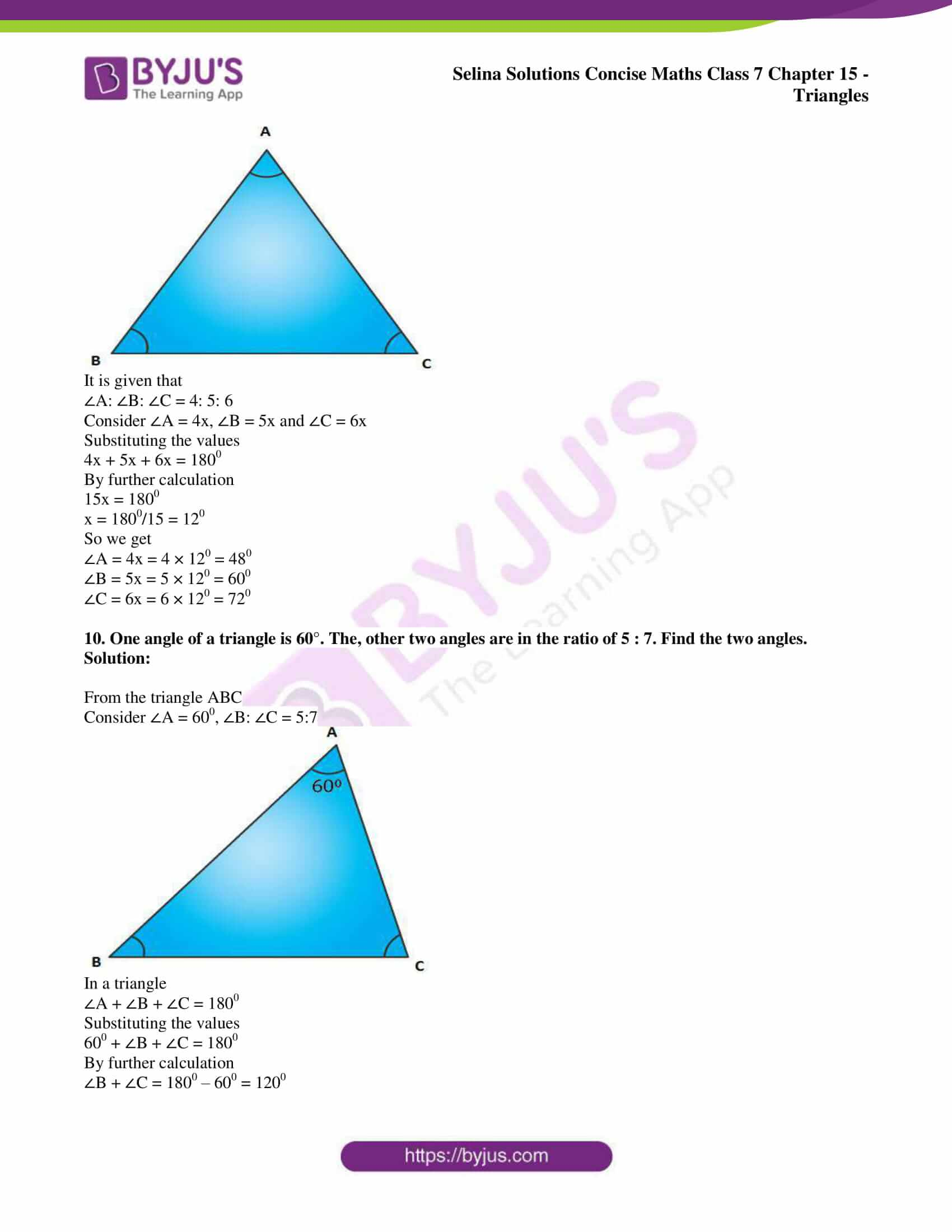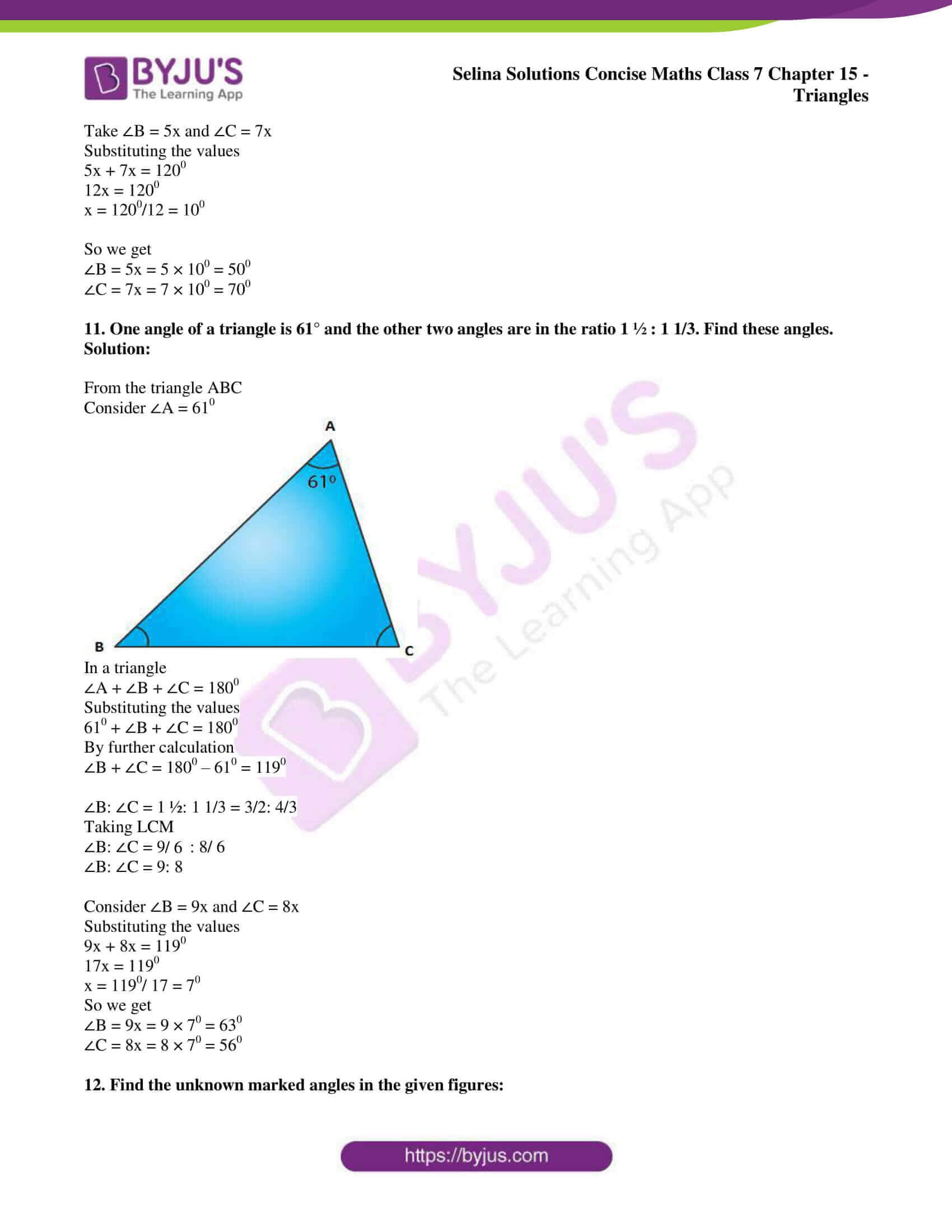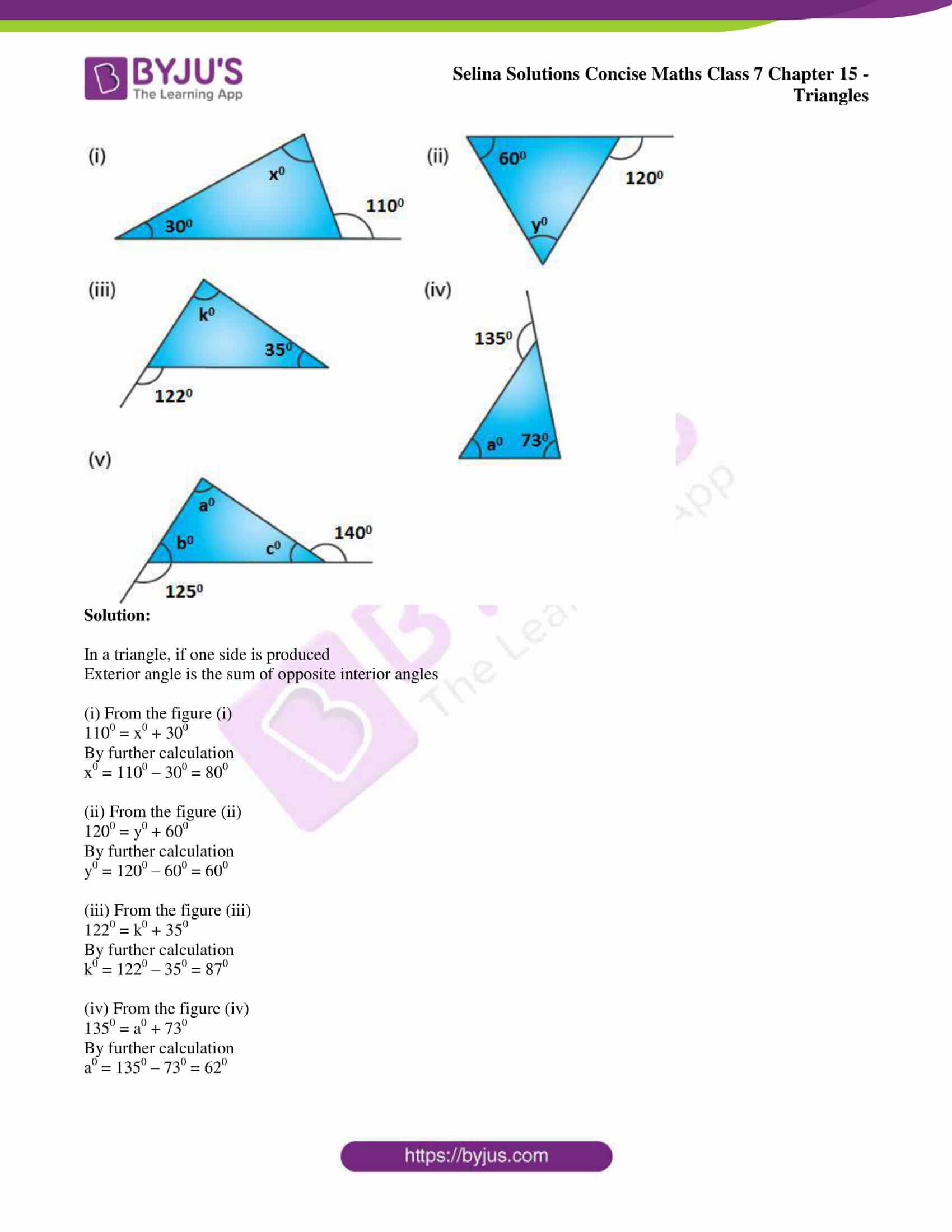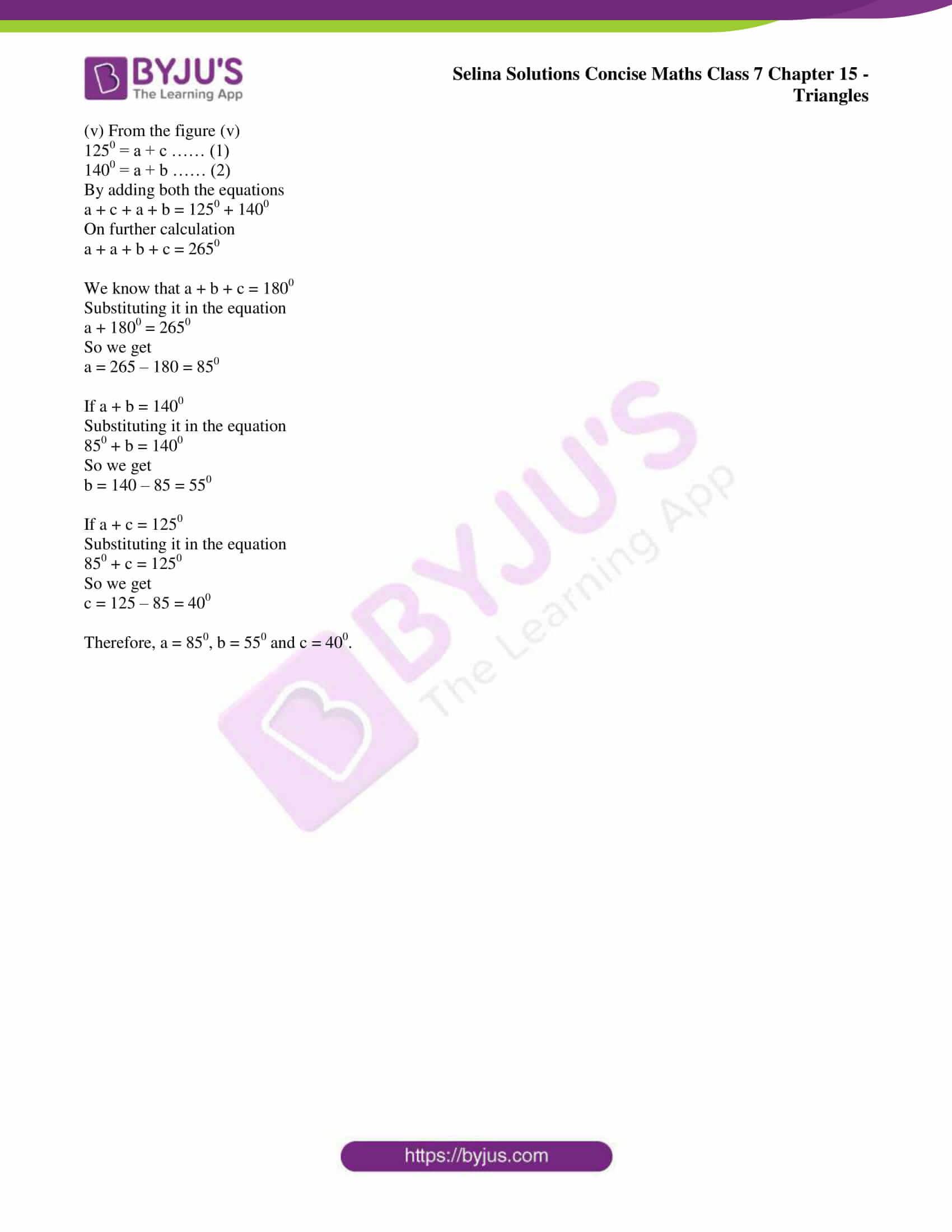### Access other exercises of Selina Solutions Concise Maths Class 7 Chapter 15: Triangles

Exercise 15B Solutions

Exercise 15C Solutions

### Access Selina Solutions Concise Maths Class 7 Chapter 15: Triangles Exercise 15A

#### Exercise 15A page: 176

1. State, if the triangles are possible with the following angles :
(i) 20°, 70° and 90°
(ii) 40°, 130° and 20°
(iii) 60°, 60° and 50°
(iv) 125°, 40° and 15°

Solution:

In a triangle, the sum of three angles is 1800

(i) 20°, 70° and 90°

Sum = 200 + 700 + 900 = 1800

Here the sum is 1800 and therefore it is possible.

(ii) 40°, 130° and 20°

Sum = 40° + 130° + 20° = 1900

Here the sum is not 1800 and therefore it is not possible.

(iii) 60°, 60° and 50°

Sum = 60° + 60° + 50° = 1700

Here the sum is not 1800 and therefore it is not possible.

(iv) 125°, 40° and 15°

Sum = 125° + 40° + 15° = 1800

Here the sum is 1800 and therefore it is possible.

2. If the angles of a triangle are equal, find its angles.

Solution:

In a triangle, the sum of three angles is 1800

So each angle = 1800/3 = 600

3. In a triangle ABC, ∠A = 45° and ∠B = 75°, find ∠C.

Solution:

In a triangle, the sum of three angles is 1800

∠A + ∠B + ∠C = 1800

Substituting the values

450 + 750 + ∠C = 1800

By further calculation

1200 + ∠C = 1800

So we get

∠C = 1800 – 1200 = 600

4. In a triangle PQR, ∠P = 60° and ∠Q = ∠R, find ∠R.

Solution:

Consider ∠Q = ∠R = x

∠P = 60°

We can write it as

∠P + ∠Q + ∠R = 1800

Substituting the values

600 + x + x = 1800

By further calculation

600 + 2x = 1800

2x = 1800 – 600 = 1200

So we get

x = 1200/2 = 600

∠Q = ∠R = 600

Therefore, ∠R = 600.

5. Calculate the unknown marked angles in each figure: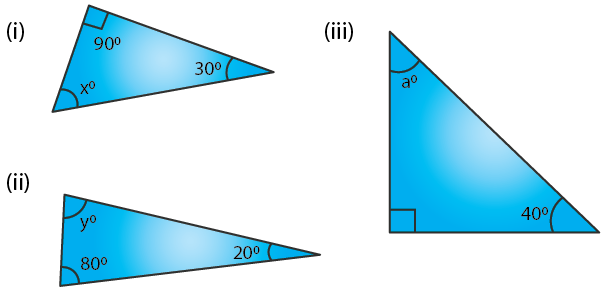Solution:

In a triangle, the sum of three angles is 1800

(i) From figure (i)

900 + 300 + x = 1800

By further calculation

1200 + x = 1800

So we get

x = 1800 – 1200 = 600

Therefore, x = 600.

(ii) From figure (ii)

y + 800 + 200 = 1800

By further calculation

y + 1000 = 1800

So we get

y = 1800 – 1000 = 800

Therefore, y = 800.

(iii) From figure (iii)

a + 900 + 400 = 1800

By further calculation

a + 1300 = 1800

So we get

a = 1800 – 1300 = 500

Therefore, a = 500.

6. Find the value of each angle in the given figures: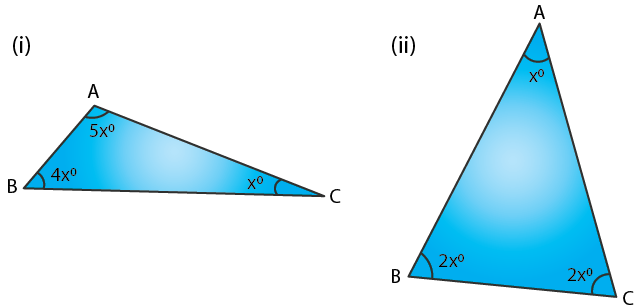Solution:

(i) From the figure (i)

∠A + ∠B + ∠C = 1800

Substituting the values

5x0 + 4x0 + x0 = 1800

By further calculation

10x0 = 1800

x = 180/10 = 180

So we get

∠A = 5x0 = 5 × 180 = 900

∠B = 4x0 = 4 × 180 = 720

∠C = x = 180

(ii) From the figure (ii)

∠A + ∠B + ∠C = 1800

Substituting the values

x0 + 2x0 + 2x0 = 1800

By further calculation

5x0 = 1800

x0 = 1800/5 = 360

So we get

∠A = x0 = 360

∠B = 2x0 = 2 × 360 = 720

∠C = 2x0 = 2 × 360 = 720

7. Find the unknown marked angles in the given figure: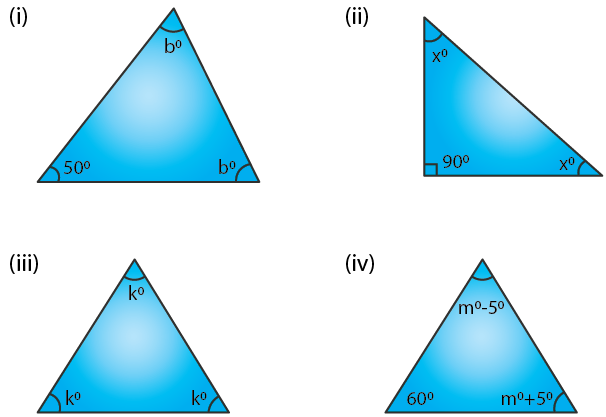Solution:

(i) From the figure (i)

∠A + ∠B + ∠C = 1800

Substituting the values

b0 + 500 + b0 = 1800

By further calculation

2b0 = 1800 – 500 = 1300

b0 = 1300/2 = 650

Therefore, ∠A = ∠C = b0 = 650.

(ii) From the figure (ii)

∠A + ∠B + ∠C = 1800

Substituting the values

x0 + 900 + x0 = 1800

By further calculation

2x0 = 1800 – 900 = 900

x0 = 900/2 = 450

Therefore, ∠A = ∠C = x0 = 450.

(iii) From the figure (iii)

∠A + ∠B + ∠C = 1800

Substituting the values

k0 + k0 + k0 = 1800

By further calculation

3k0 = 1800

k0 = 1800/3 = 600

Therefore, ∠A = ∠B = ∠C = 600.

(iv) From the figure (iv)

∠A + ∠B + ∠C = 1800

Substituting the values

(m0 – 50) + 600 + (m0 + 50) = 1800

By further calculation

m0 – 50 + 600 + m0 + 50 = 1800

2m0 = 1800 – 600 = 1200

m0 = 1200/2 = 600

Therefore, ∠A = m0 – 50 = 600 – 50 = 550

∠C = m0 + 50 = 600 + 50 = 650

8. In the given figure, show that: ∠a = ∠b + ∠c

(i) If ∠b = 60° and ∠c = 50°; find ∠a.
(ii) If ∠a = 100° and ∠b = 55°; find ∠c.
(iii) If ∠a = 108° and ∠c = 48°; find ∠b.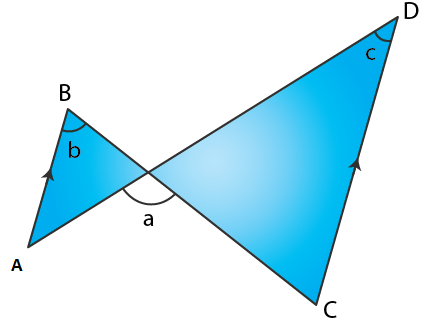Solution:

From the figure

AB || CD

b = ∠C and ∠A = c are alternate angles

In triangle PCD

Exterior ∠APC = ∠C + ∠D

a = b + c

(i) If ∠b = 60° and ∠c = 50°

∠a = ∠b + ∠c

Substituting the values

∠a = 60 + 50 = 1100

(ii) If ∠a = 100° and ∠b = 55°

∠a = ∠b + ∠c

Substituting the values

∠c = 100 – 55 = 450

(iii) If ∠a = 108° and ∠c = 48°

∠a = ∠b + ∠c

Substituting the values

∠b = 108 – 48 = 600

9. Calculate the angles of a triangle if they are in the ratio 4 : 5 : 6.

Solution:

In a triangle, the sum of angles of a triangle is 1800

∠A + ∠B + ∠C = 1800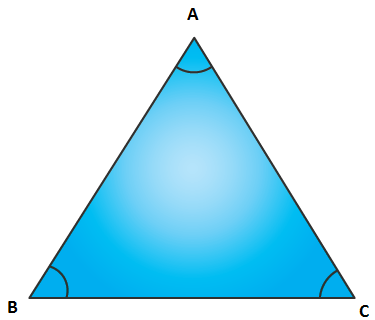It is given that

∠A: ∠B: ∠C = 4: 5: 6

Consider ∠A = 4x, ∠B = 5x and ∠C = 6x

Substituting the values

4x + 5x + 6x = 1800

By further calculation

15x = 1800

x = 1800/15 = 120

So we get

∠A = 4x = 4 × 120 = 480

∠B = 5x = 5 × 120 = 600

∠C = 6x = 6 × 120 = 720

10. One angle of a triangle is 60°. The, other two angles are in the ratio of 5 : 7. Find the two angles.

Solution:

From the triangle ABC

Consider ∠A = 600, ∠B: ∠C = 5:7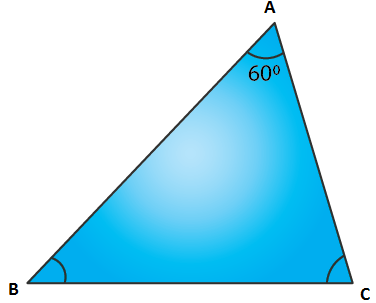In a triangle

∠A + ∠B + ∠C = 1800

Substituting the values

600 + ∠B + ∠C = 1800

By further calculation

∠B + ∠C = 1800 – 600 = 1200

Take ∠B = 5x and ∠C = 7x

Substituting the values

5x + 7x = 1200

12x = 1200

x = 1200/12 = 100

So we get

∠B = 5x = 5 × 100 = 500

∠C = 7x = 7 × 100 = 700

11. One angle of a triangle is 61° and the other two angles are in the ratio 1 ½ : 1 1/3. Find these angles.

Solution:

From the triangle ABC

Consider ∠A = 610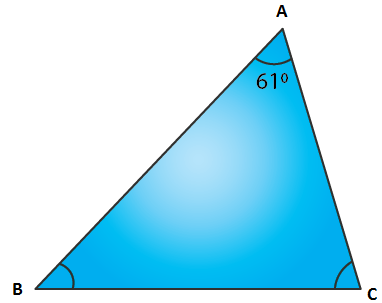In a triangle

∠A + ∠B + ∠C = 1800

Substituting the values

610 + ∠B + ∠C = 1800

By further calculation

∠B + ∠C = 1800 – 610 = 1190

∠B: ∠C = 1 ½: 1 1/3 = 3/2: 4/3

Taking LCM

∠B: ∠C = 9/6: 8/ 6

∠B: ∠C = 9: 8

Consider ∠B = 9x and ∠C = 8x

Substituting the values

9x + 8x = 1190

17x = 1190

x = 1190/ 17 = 70

So we get

∠B = 9x = 9 × 70 = 630

∠C = 8x = 8 × 70 = 560

12. Find the unknown marked angles in the given figures: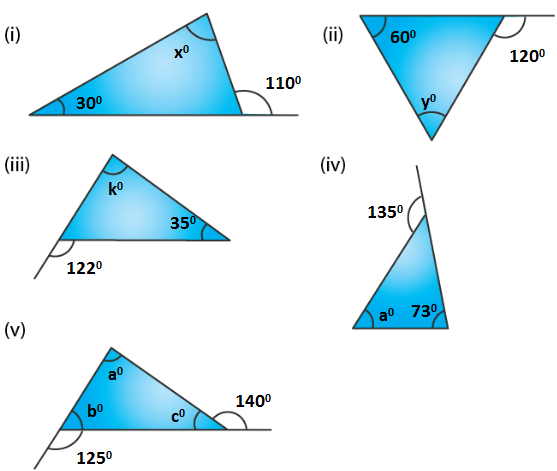Solution:

In a triangle, if one side is produced

Exterior angle is the sum of opposite interior angles

(i) From the figure (i)

1100 = x0 + 300

By further calculation

x0 = 1100 – 300 = 800

(ii) From the figure (ii)

1200 = y0 + 600

By further calculation

y0 = 1200 – 600 = 600

(iii) From the figure (iii)

1220 = k0 + 350

By further calculation

k0 = 1220 – 350 = 870

(iv) From the figure (iv)

1350 = a0 + 730

By further calculation

a0 = 1350 – 730 = 620

(v) From the figure (v)

1250 = a + c …… (1)

1400 = a + b …… (2)

a + c + a + b = 1250 + 1400

On further calculation

a + a + b + c = 2650

We know that a + b + c = 1800

Substituting it in the equation

a + 1800 = 2650

So we get

a = 265 – 180 = 850

If a + b = 1400

Substituting it in the equation

850 + b = 1400

So we get

b = 140 – 85 = 550

If a + c = 1250

Substituting it in the equation

850 + c = 1250

So we get

c = 125 – 85 = 400

Therefore, a = 850, b = 550 and c = 400.